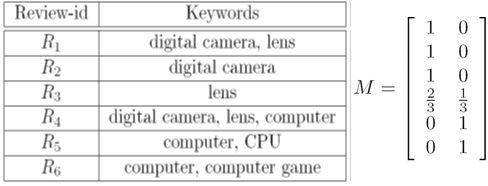# Current Slide

Small screen detected. You are viewing the mobile version of SlideWiki. If you wish to edit slides you will need to use a larger device.

### Fuzzy (Soft) Clustering

 Example: Let cluster feature beC1: “digital camera” & “lense”C2: “computer”• Fuzzy clustering:
• k fuzzy clusters C1, …, Ck, represented as a partition matrix M = [wij]
• P1: For each object oi and cluster Cj, 0 ≤ wij ≤ 1(fuzzy set)
• P2: For each object oi,
$\sum_{i=1}^{k} w_{ij}=1$
(equal participation in clustering)
• P3: For each cluster Cj,
$0<\sum_{i=1}^{n} w_{ij} (ensure no empty cluster) • Let c1, …, ck as the centers of k clusters • For each object oi, sum of square error SSE, p is a parameter: \[SSE(C_{j})=\sum_{i=1}^{n} w_{ij}^{p}dist(o_{i},c_{j})^2$

$SSE(o_{j})=\sum_{j=1}^{k} w_{ij}^{p}dist(o_{i},c_{j})^2$

• For each cluster C, sum of square error:$SSE(C)=\sum_{i=1}^{n} \sum_{j=1}^{k} w_{ij}^{p}dist(o_{i},c_{j})^2$

Speaker notes:

## Content Tools

### Sources

There are currently no sources for this slide.